# 可视化模块搭建交易策略--进阶

### 交易类别模块

1、获取交易所个数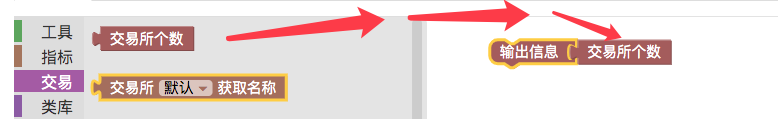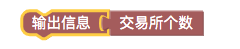``````function main () {
Log(exchanges.length)
}
``````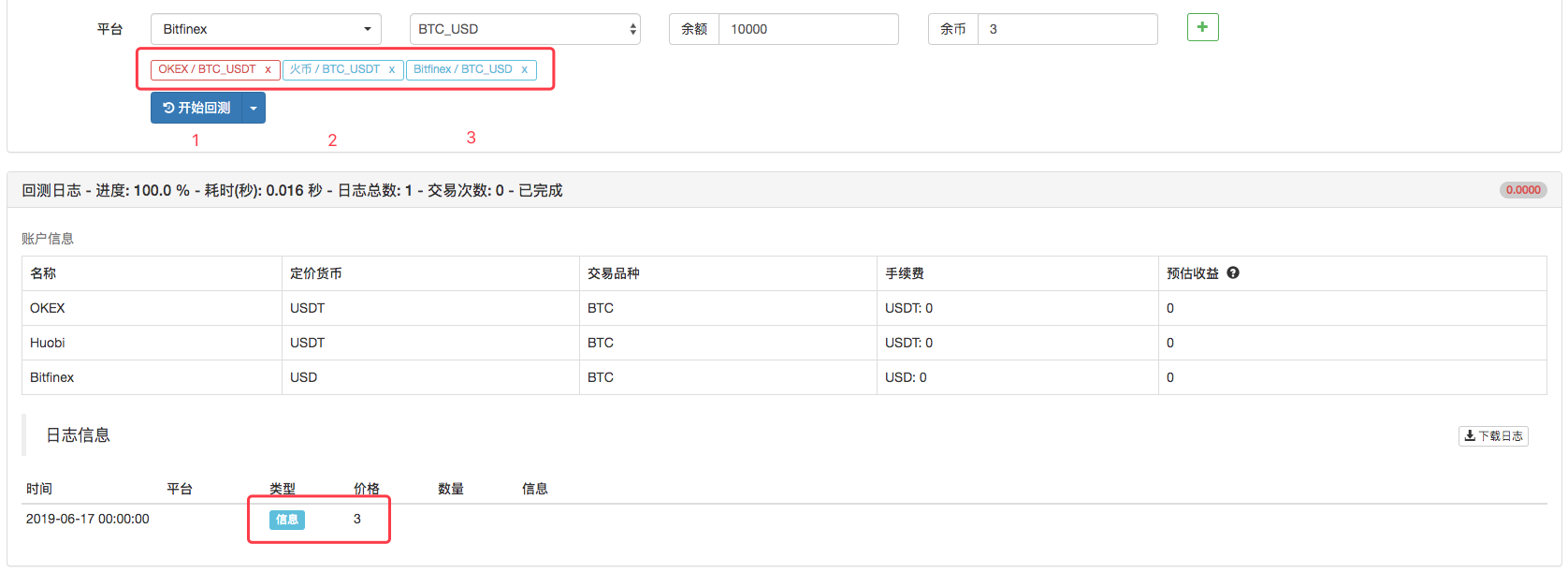2、获取交易所名称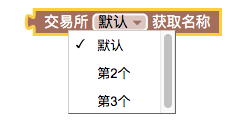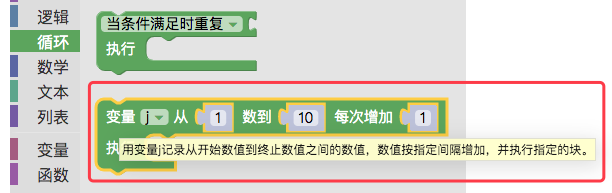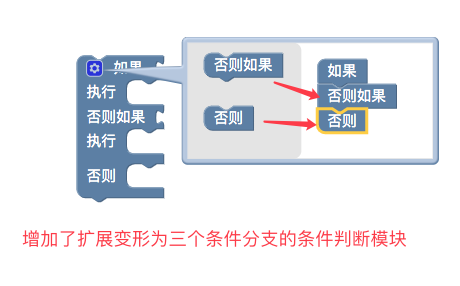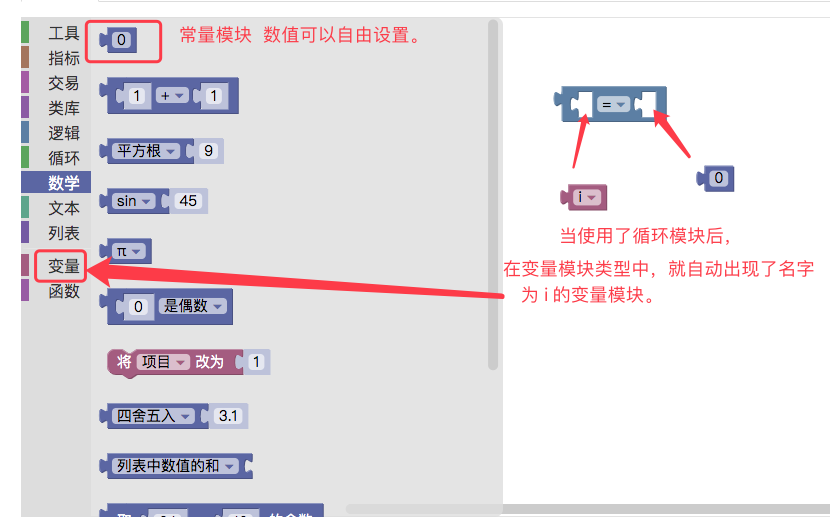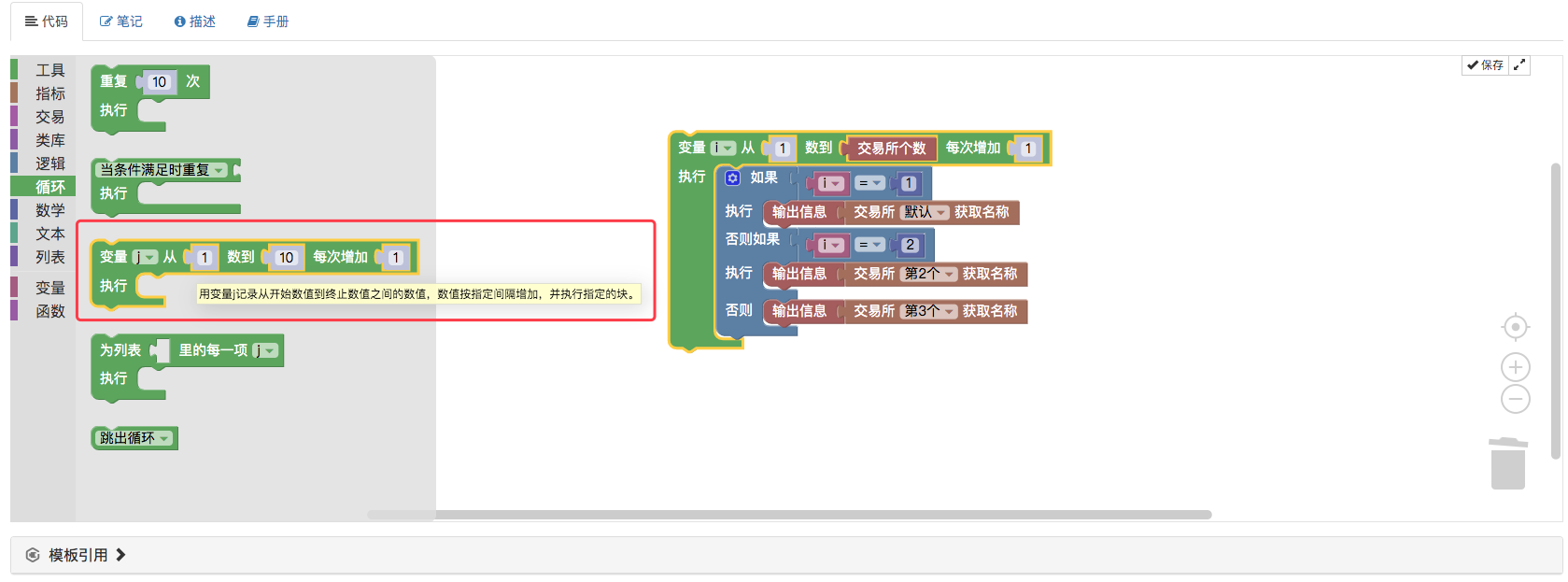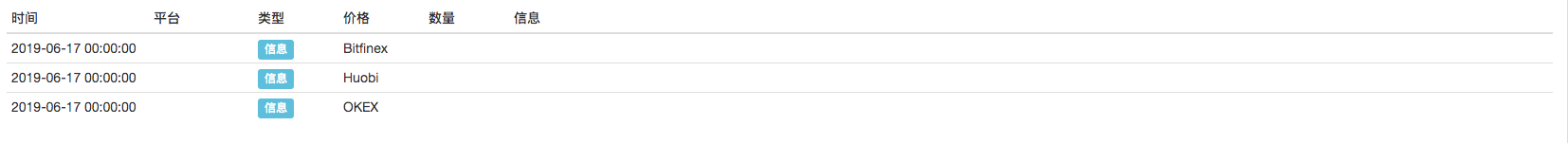``````function main () {
for (var i = 1 ; i <= exchanges.length ; i++) {
if (i == 1) {
Log(exchanges.GetName())
} else if (i == 2) {
Log(exchanges.GetName())
} else {
Log(exchanges.GetName())
}
}
}
``````

3、获取交易所当前交易对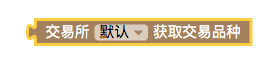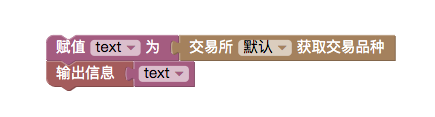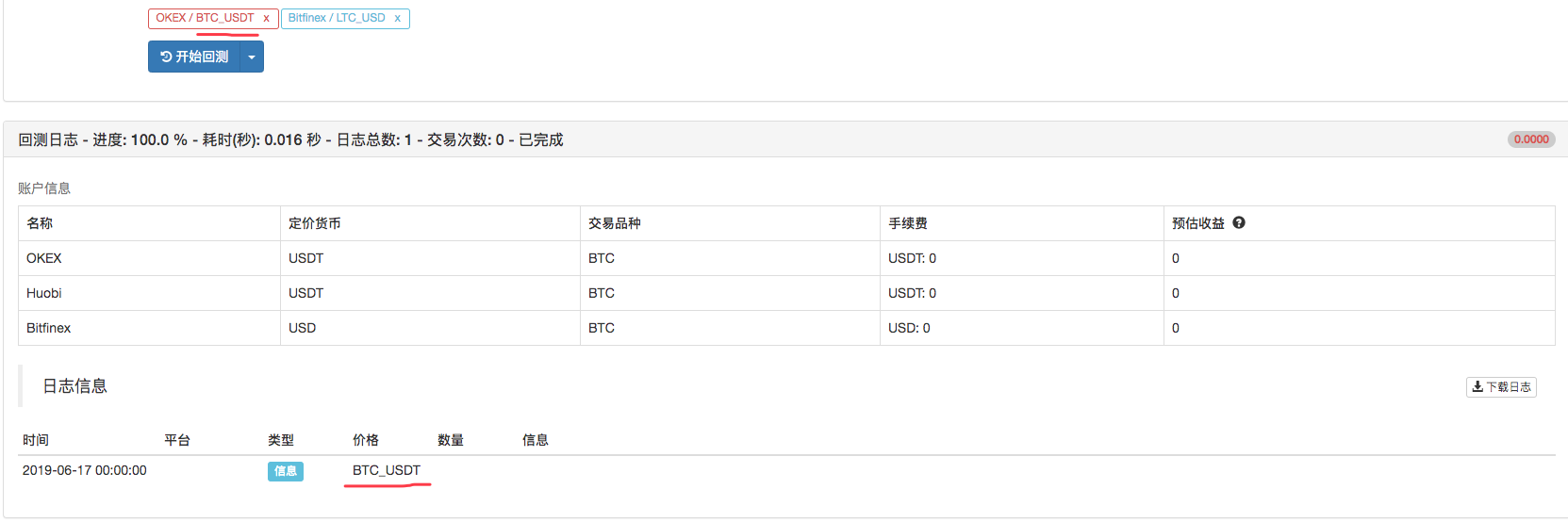``````function main () {
var text = exchange.GetCurrency()
Log(text)
}
``````

4、下单模块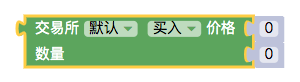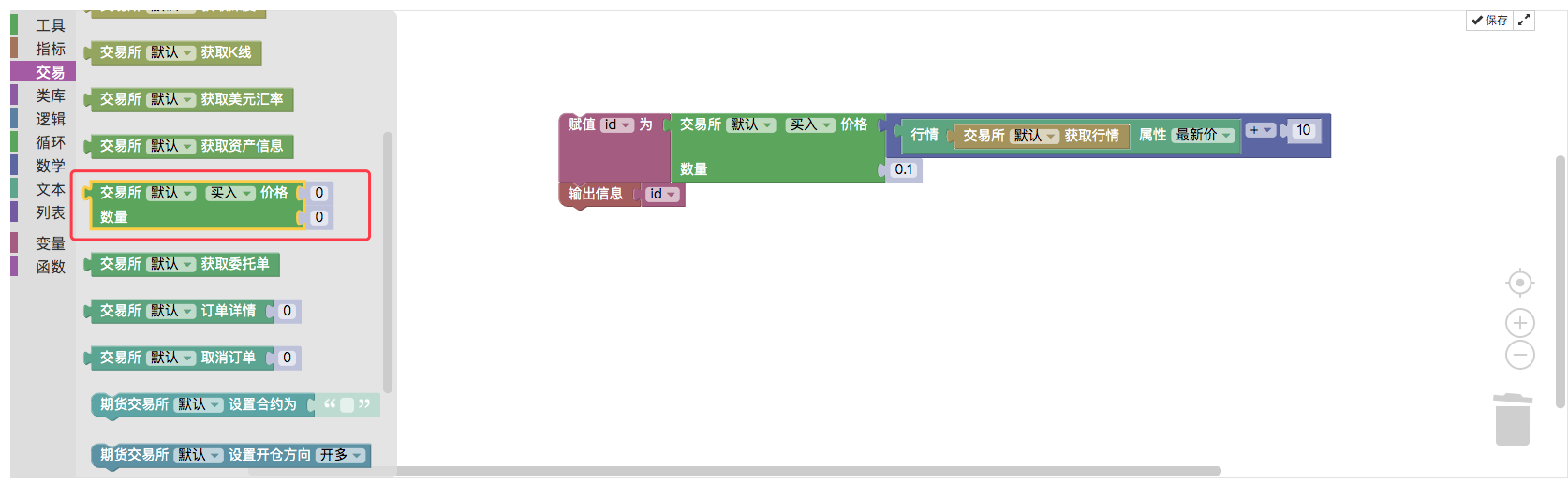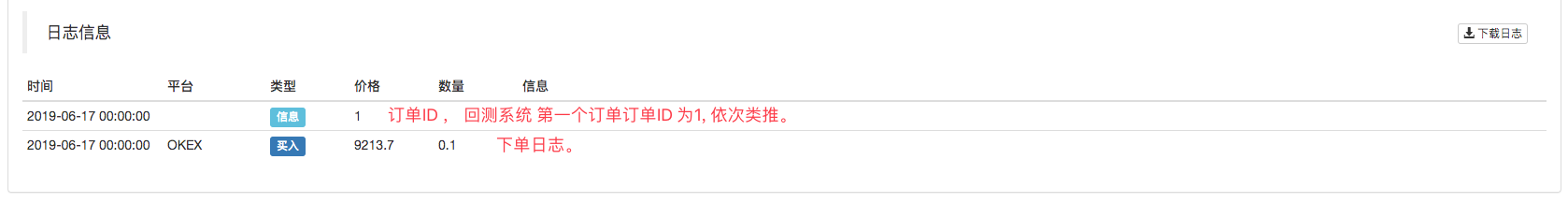``````function main () {
var id = exchange.Buy(_C(exchange.GetTicker).Last + 10, 0.1)
Log(id)
}
``````

5、获取当前交易对委托订单的模块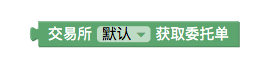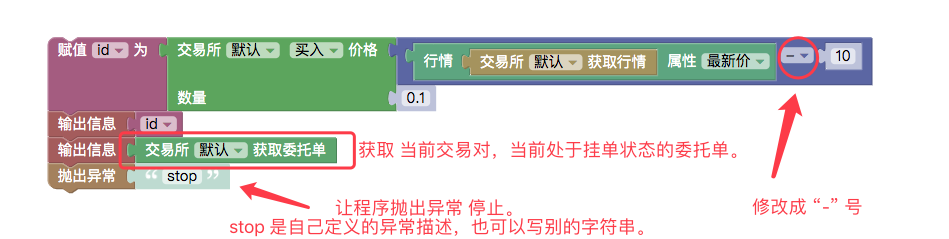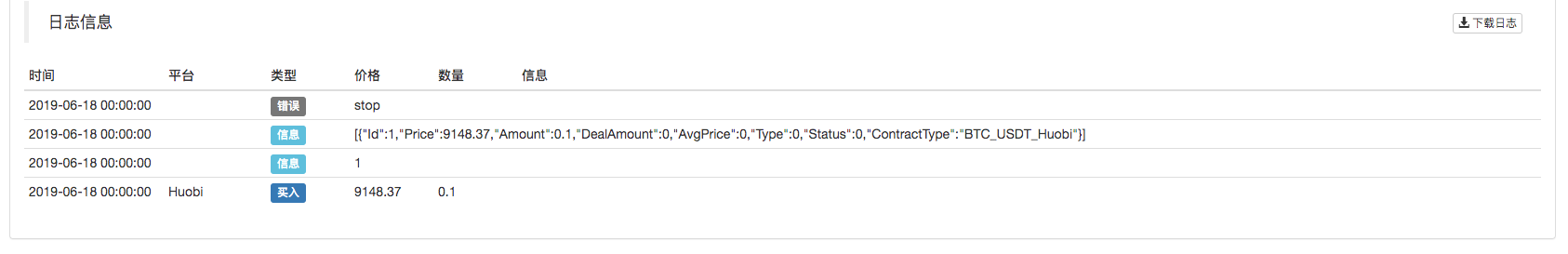``````function main () {
var id = exchange.Buy(_C(exchange.GetTicker).Last - 10, 0.1)
Log(id)
Log(exchange.GetOrders())
throw "stop"
}
``````

6、取消订单模块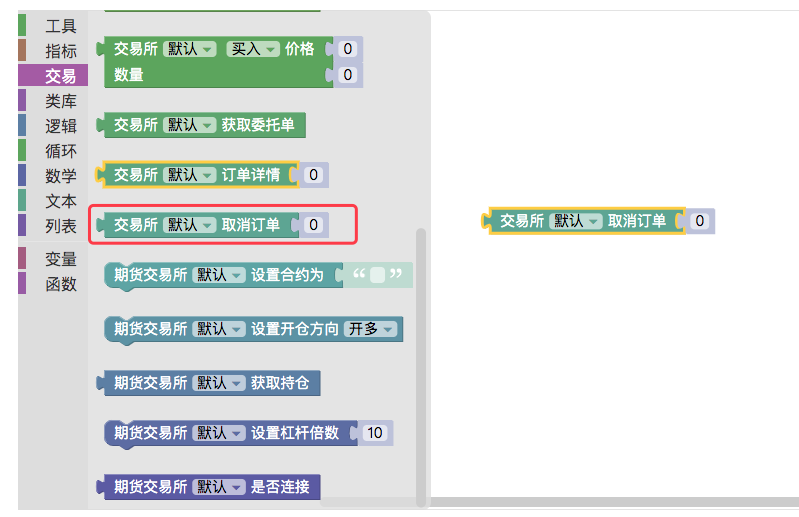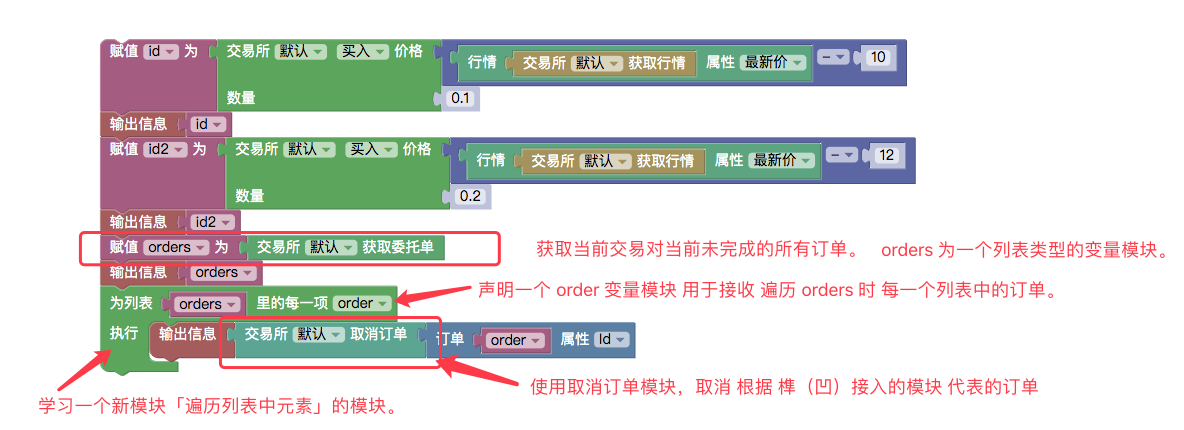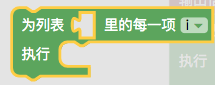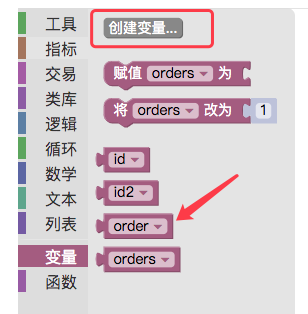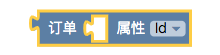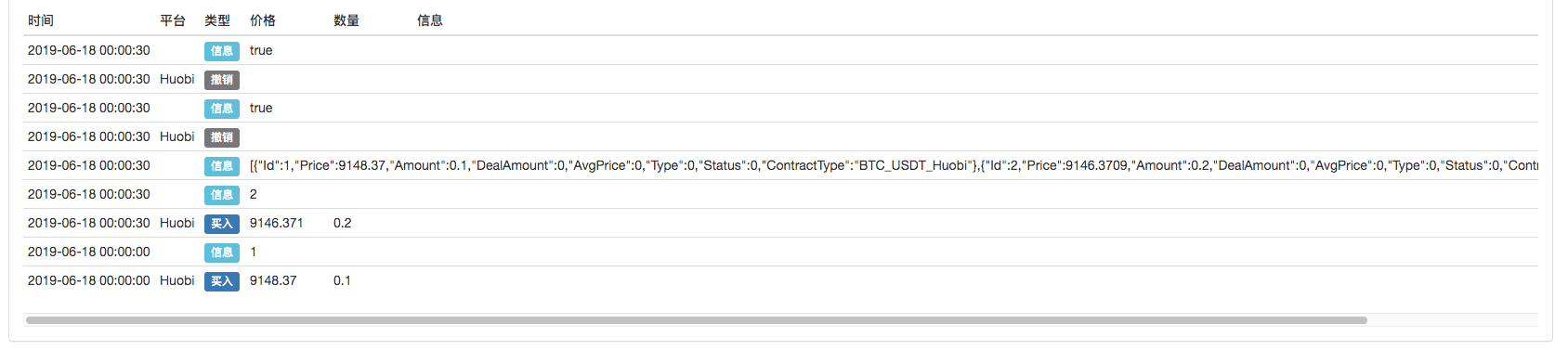``````function main () {
var id = exchange.Buy(_C(exchange.GetTicker).Last - 10, 0.1)
Log(id)
var id2 = exchange.Buy(_C(exchange.GetTicker).Last - 12, 0.2)
Log(id2)
var orders = exchange.GetOrders()
Log(orders)
for (var i in orders) {
var order = orders[i]
Log(exchange.CancelOrder(order.Id))
}
}
``````

7、根据订单ID获取某个订单详细信息的模块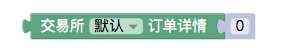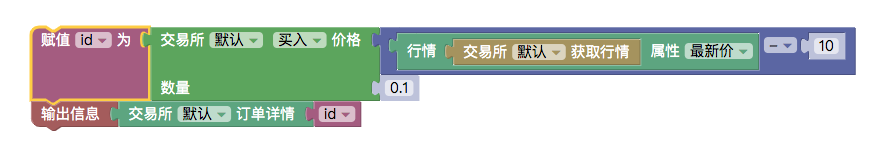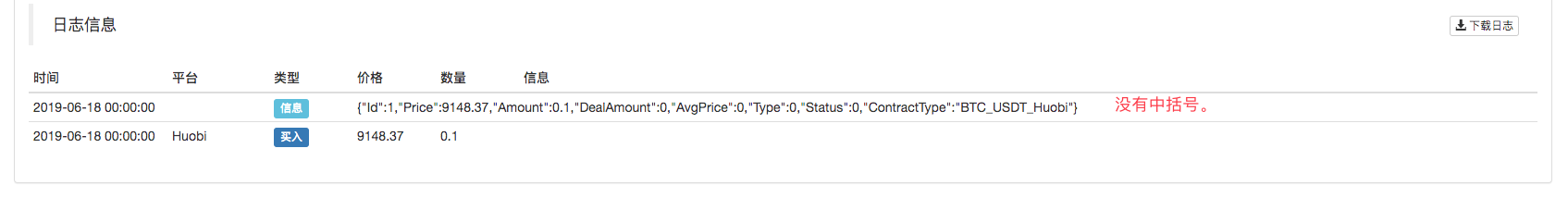``````function main () {
var id = exchange.Buy(_C(exchange.GetTicker).Last - 10, 0.1)
Log(exchange.GetOrder(id))
}
``````

8、期货交易模块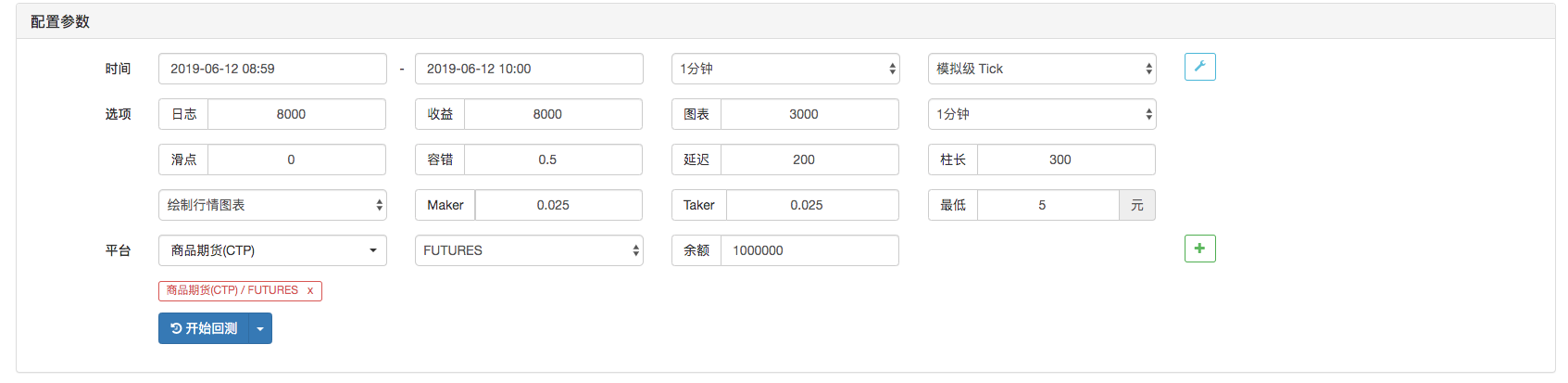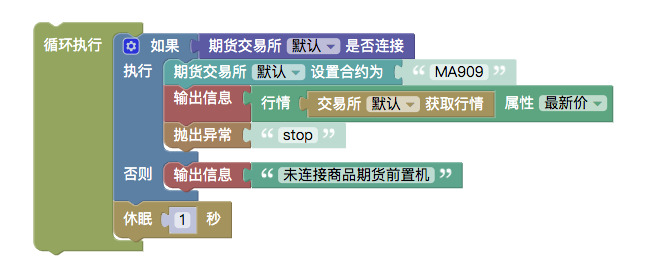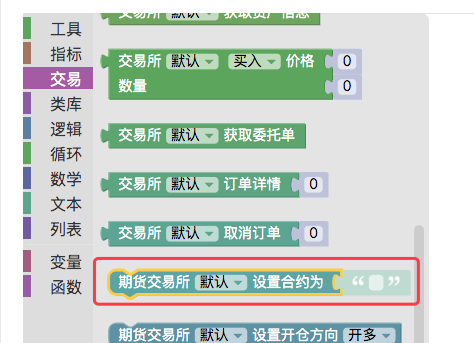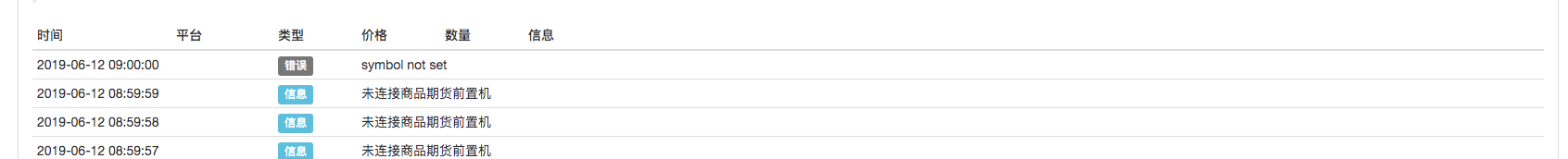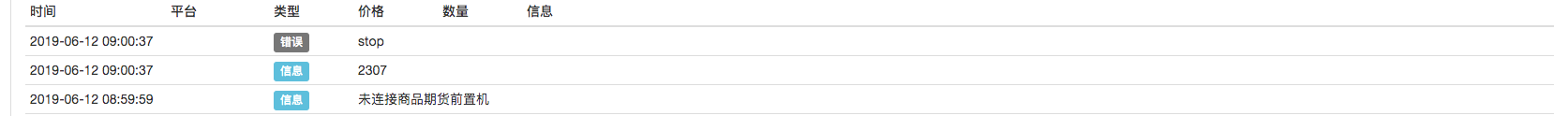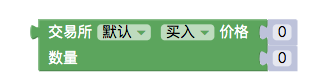• sell : 开空仓
• closesell : 平空仓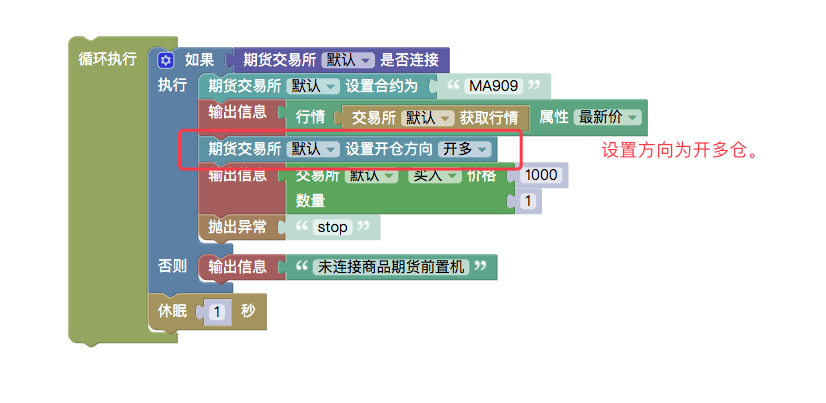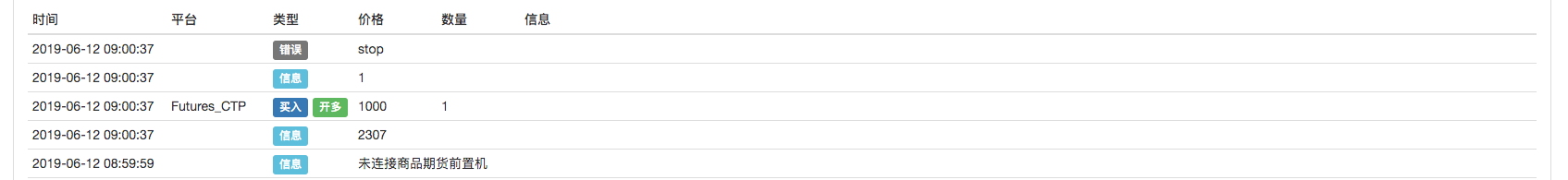``````function main () {
while (true) {
if (exchange.IO("status")) {
exchange.SetContractType("MA909")
Log(exchange.GetTicker().Last)
throw "stop"
} else {
Log("未连接商品期货前置机")
}
Sleep(1000)
}
}
``````

9、数字货币期货交易模块

• 合约代码以OKEX为例 可以是：

• this_week : 当周
• next_week : 次周
• quarter : 季度
• swap : 永续
• BitMEX :

• XBTUSD
• ETHUSD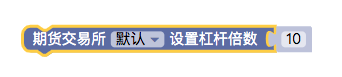``````# 注意 ： 回测不支持。
``````

``````function main () {
exchange.SetMarginLevel(10)
}
``````